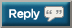# Thread: 2017 Push-up Challenge - try for 40,000 this time

1. 10,910 + 50 = 10,9602.3.4.5.6. adding only the ones ive tracked +104= 11,4027. adding only the ones ive tracked +104= 11,402
11,402 + 80 = 11,4828. 11,482 + 150 = 11,6329. from yesterday:

11,632 + 80 = 11,71210. 11712 + 20= 1173211. This is truly amazing ! Great job everyone!12.13.14.15.16. + 50 = 1195517.18.19. + 24 = 12,29420. From Saturday, had to take a break yesterday....jobs get in the way of doing fun stuff

12,294 + 40 = 12,33421.22.23. 12,969 + 40 = 13,00924.25.26. 13152 + 30 = 1318227.28.29.30. 13,372 + 100 = 13,472#### Posting Permissions

• You may not post new threads
• You may not post replies
• You may not post attachments
• You may not edit your posts